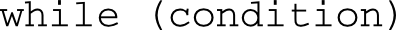# 5.5 - while 陳述

 1. 控制變數初始設定 2. 迴圈結束條件測試 3. 調整控制變數的值``````#include <iostream>

int main() {
int sum = 0;
int i = 1;

while (i <= 100) {
sum += i;
i++;
}

std::cout << "1 + ... +  100 = " << sum << std::endl;

return 0;
}

/* 《程式語言教學誌》的範例程式
http://kaiching.org/
檔名：u0505_1.cpp
功能：示範 C++ 的 while 陳述
作者：張凱慶*/``````

 \$ g++ u0505_1.cpp \$ ./a.out 1 + ... + 100 = 5050 \$

``````#include <iostream>

int main() {
int i = 1;
int j = 1;

while (i <= 9) {
while (j <= 9) {
std::cout << i * j << " ";
j++;
}

j = 1;
i++;
std::cout << std::endl;
}

return 0;
}

/* 《程式語言教學誌》的範例程式
http://kaiching.org/
檔名：u0505_2.cpp
功能：示範 C++ 的 while 陳述
作者：張凱慶*/``````

 g++ u0505_2.cpp \$ ./a.out 1 2 3 4 5 6 7 8 9 2 4 6 8 10 12 14 16 18 3 6 9 12 15 18 21 24 27 4 8 12 16 20 24 28 32 36 5 10 15 20 25 30 35 40 45 6 12 18 24 30 36 42 48 54 7 14 21 28 35 42 49 56 63 8 16 24 32 40 48 56 64 72 9 18 27 36 45 54 63 72 81 \$

while 迴圈也可利用 for 迴圈來取代，反之亦然。